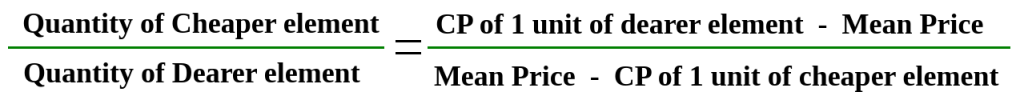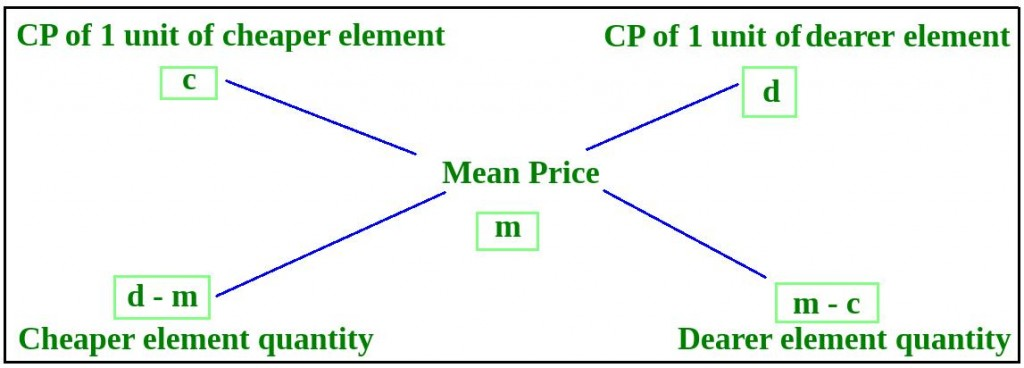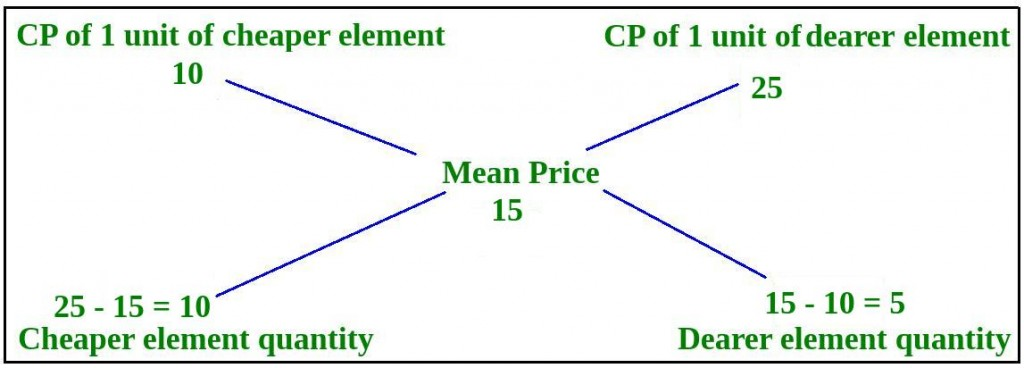Open In App
Related Articles

# Alligation or Mixture – Aptitude Questions and Answers

Mixture and alligation are important concepts in quantitative aptitude used to determine the ratio and cost of sale for a mixture made up of two or more materials. In competitive exams, mixture and alligation questions are commonly asked to test a candidate’s ability to calculate the mean value of a mixture with different ratios and amounts of ingredients, as well as to find the proportions of elements being mixed.

While these concepts may seem challenging, regular practice and solving similar questions can help candidates gain insight and clarity into this topic. To prepare for these types of questions, it’s important to familiarize oneself with the quantitative aptitude syllabus and the exams in which this section is included.

Practice Quiz :

## Mixture and Alligation: Concept and Formulas

• Mixture is a combination of two or more elements to form a third element. The questions from mixture are usually based on Ratio and Proportion and do not require any special formula.
• Alligation is a rule that enables us to quickly calculate the price of a mixture, given that it is a mix of two elements having different prices.
• Alligation Rule :•  Here, mean price is the price of 1 unit of the mixture. Please note that in the above formula, everything has to be calculated by considering one unit only. Another point to be noted is that mean price would always be between cheaper price and dearer price.
• In questions of replacement where we initially have some quantity of pure element (like petrol), and we keep on replacing a fixed portion of this pure liquid every time with some other element (like water) thus making it a mixture, we apply the following formula for finding the quantity of pure element after ‘n’ replacements : P x [1 – (R / P)]n, where P is the initial quantity of pure element R is the quantity replaced every time n is the number of replacement

## Tips and Tricks to Solve Mixture and Alligation Questions

• The alligation rule is a mixture problem-solving technique that can be used to calculate the rate at which an article can be sold.
• By using this technique, one can figure out the ratio between two different ingredients in a given mixture.
• Questions from topics such as partnerships, time and work, and wages can also be solved easily with this concept.
• It may seem complicated at first but once you get familiar with the formulas involved, it becomes much simpler.

## Sample Questions on Mixture and Alligation

### Q1: From a vessel of 20 liters of pure milk, 1 liter is taken out and replaced with water, so as to keep the volume constant at 20 liters. This process is repeated 5 times. Find the percentage of pure milk left in the vessel after 5 replacements.

Solution:

Here, we need to apply the formula P x [1 – (R / P)]n P = Initial quantity of pure element = 20 liters R = Quantity replaced every time = 1-liter n = Number of replacements = 5 So, the quantity of pure milk after 5 replacements = 20 x [1 – (1 / 20)]5 Quantity of pure milk after 5 replacements = 20 x (0.95)5 = 20 x 0.773780938 = 15.4756 liters Therefore, percentage of pure milk left in the vessel after 5 replacements = (15.4756 / 20) x 100 = 77.378 %

### Q2: A dishonest shopkeeper mixed cheaper quality rice, priced at Rs. 10 / KG with good quality rice, priced at Rs. 25 / KG, and sells the mixture at Rs. 15 / KG. Find the ratio in which he mixes the two qualities of rice.

Solution:

Thus, the ratio of quantities of cheaper and good quality rice = 10: 5 = 2: 1### Q3: A container contains a mixture of two liquids P and Q in the ratio of 7:5. When 9 liters of mixture is taken out and replaced with Q, the ratio becomes 7:9. Find the quantity of liquid P in the container.

Solution:

Let the quantity of mixture in the container be 12x liters (i.e., 7x liters of liquid P and 5x liters of liquid Q). Then, after the first replacement, the quantity of mixture in the container = 12x – 9 liters (i.e., 7x – (9/12) x 7x + (9/12) x 5x liters of liquid P and 5x – (9/12) x 5x + (9/12) x 7x liters of liquid Q).
The ratio of liquid P to liquid Q in the final mixture = 7x – (9/12) x 7x + (9/12) x 5x : 5x – (9/12) x 5x + (9/12) x 7x = 7:9.
Solving the above equation, we get x = 36 liters.
Therefore, the quantity of liquid P in the container = 7x = 252 liters.

## Related Articles:

Problems on Mixture and Alligation | Set 2

Test your knowledge of Mixture and Alligation in Quantitative Aptitude with the quiz linked below, containing numerous practice questions to help you master the topic:-

## FAQs on Mixture and Alligation

### Q1. What are alligation or mixture questions in quantitative aptitude?

Solution:

Alligation or mixture questions in quantitative aptitude are those that involve finding the ratio in which two or more components with different prices or strengths are mixed together to get a required strength or price.

### Q3. What are some common strategies or formulas used to solve alligation or mixture questions?

Solution:

Some common formulas used to solve alligation or mixture questions include the rule of alligation, the weighted average formula, and the proportion formula.

### Q4. What are some examples of alligation or mixture questions commonly asked in competitive exams?

Solution:

Here’s an example:

A chemist has a 20% alcohol solution and a 60% alcohol solution. How many liters of each should be mixed together to make 100 liters of a 40% alcohol solution?